/ 猿问

# 如何在OpenCV中定义分水岭的标记？

2019-10-23 16:59:34

`minMaxLoc()`会给我带来价值，但是如何将其限制在我感兴趣的斑点上？我可以利用来自`findContours()`或cvBlob Blob 的结果来限制ROI并将最大值应用于每个Blob吗？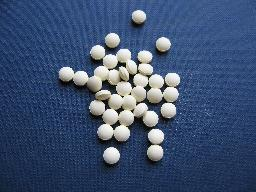## 3 回答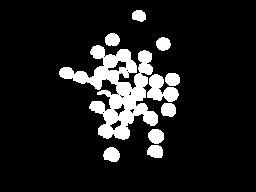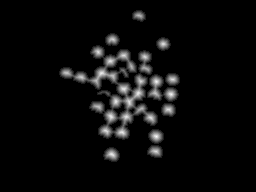根据距离变换结果，我们可以考虑一些阈值，以便仅考虑距背景最远的区域（下图为左图）。这样做，通过在较早的阈值之后标记不同的区域，我们可以获得每个对象的标记。现在，我们还可以考虑将左侧图片的放大版本的边框组成标记。完整的标记显示在右侧的下方（某些标记太暗而看不见，但左侧图像中的每个白色区域均在右侧图像中表示）。import sys

import cv2

import numpy

from scipy.ndimage import label

def segment_on_dt(a, img):

border = cv2.dilate(img, None, iterations=5)

border = border - cv2.erode(border, None)

dt = cv2.distanceTransform(img, 2, 3)

dt = ((dt - dt.min()) / (dt.max() - dt.min()) * 255).astype(numpy.uint8)

_, dt = cv2.threshold(dt, 180, 255, cv2.THRESH_BINARY)

lbl, ncc = label(dt)

lbl = lbl * (255 / (ncc + 1))

# Completing the markers now.

lbl[border == 255] = 255

lbl = lbl.astype(numpy.int32)

cv2.watershed(a, lbl)

lbl[lbl == -1] = 0

lbl = lbl.astype(numpy.uint8)

return 255 - lbl

# Pre-processing.

img_gray = cv2.cvtColor(img, cv2.COLOR_BGR2GRAY)

_, img_bin = cv2.threshold(img_gray, 0, 255,

cv2.THRESH_OTSU)

img_bin = cv2.morphologyEx(img_bin, cv2.MORPH_OPEN,

numpy.ones((3, 3), dtype=int))

result = segment_on_dt(img, img_bin)

cv2.imwrite(sys.argv, result)

result[result != 255] = 0

result = cv2.dilate(result, None)

img[result == 255] = (0, 0, 255)

cv2.imwrite(sys.argv, img)1-首先，我们加载图像，将其转换为灰度，并使用合适的值对其进行阈值处理。我进行了Otsu的二值化，因此它将找到最佳阈值。

import cv2

import numpy as np

gray = cv2.cvtColor(img,cv2.COLOR_BGR2GRAY)

ret,thresh = cv2.threshold(gray,0,255,cv2.THRESH_BINARY+cv2.THRESH_OTSU)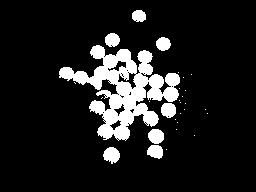（即使结果很好，因为前景和背景图像之间的对比度很高）

2-现在我们必须创建标记。标记是具有与原始图像相同大小的图像，即32SC1（32位带符号单通道）。

A-前景区域：-我们已经有了一个阈值图像，其中药丸是白色的。我们会稍微侵蚀它们，以确保其余区域属于前景。

fg = cv2.erode(thresh,None,iterations = 2)

fg：B-背景区域：-在这里我们扩大阈值图像，以便减少背景区域。但是我们确定剩下的黑色区域是100％背景。我们将其设置为128。

bgt = cv2.dilate(thresh,None,iterations = 3)

ret,bg = cv2.threshold(bgt,1,128,1)C-现在我们同时添加fg和bg：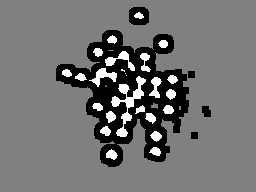marker32 = np.int32(marker)

3-最后，我们应用分水岭并将结果转换回uint8图片：

cv2.watershed(img,marker32)

m = cv2.convertScaleAbs(marker32)4 - 我们的门槛得当拿到面具，并执行bitwise_and与输入图像：

ret,thresh = cv2.threshold(m,0,255,cv2.THRESH_BINARY+cv2.THRESH_OTSU)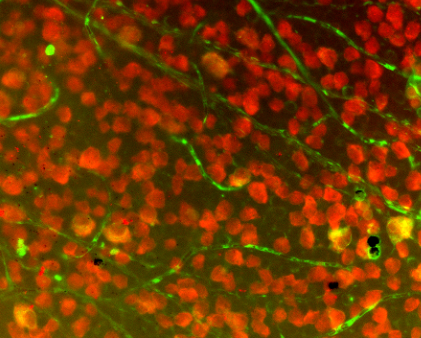1. 从源图像开始（左图，用于演示）

2. 隔离红色通道（左中间）

3. 应用自适应阈值（右中间）

4. 找到轮廓，然后消除那些面积较小的轮廓（右）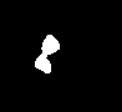var isolatedContour = new Mat(source.Size(), MatType.CV_8UC1, new Scalar(0, 0, 0));

Cv2.DrawContours(isolatedContour, new List<List<Point>> { contour }, -1, new Scalar(255, 255, 255), -1);var kernel = Cv2.GetStructuringElement(MorphShapes.Ellipse, new Size(2, 2));

var background = new Mat();

Cv2.Dilate(isolatedContour, background, kernel, iterations: 8);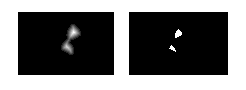var foreground = new Mat(source.Size(), MatType.CV_8UC1);

Cv2.Normalize(foreground, foreground, 0, 1, NormTypes.MinMax); //Remember to normalize!

foreground.ConvertTo(foreground, MatType.CV_8UC1, 255, 0);

Cv2.Threshold(foreground, foreground, 150, 255, ThresholdTypes.Binary);

var unknown = new Mat(); //this variable is also named "border" in some examples

Cv2.Subtract(background, foreground, unknown);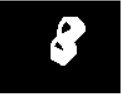0 0 0 0 0 0 0 0 0

0 1 0 1 0 2 2 2 0

0 1 0 1 0 0 2 0 0

0 1 1 1 0 0 2 0 0

0 1 0 1 0 0 2 0 0

0 1 0 1 0 2 2 2 0

0 0 0 0 0 0 0 0 0

var labels = new Mat(); //also called "markers" in some examples

Cv2.ConnectedComponents(foreground, labels);

labels = labels + 1;

//this is a much more verbose port of numpy's: labels[unknown==255] = 0

for (int x = 0; x < labels.Width; x++)

{

for (int y = 0; y < labels.Height; y++)

{

//You may be able to just send "int" in rather than "char" here:

var labelPixel = (int)labels.At<char>(y, x);    //note: x and y are inexplicably

var borderPixel = (int)unknown.At<char>(y, x);  //and infuriatingly reversed

if (borderPixel == 255)

labels.Set(y, x, 0);

}

}

Cv2.Dilate(isolatedContour, mask, new Mat(), iterations: 20);

var sourceCrop = new Mat(source.Size(), source.Type(), new Scalar(0, 0, 0));

Cv2.Watershed(sourceCrop, labels);

var watershedContours = new List<Tuple<int, List<Point>>>();

for (int x = 0; x < labels.Width; x++)

{

for (int y = 0; y < labels.Height; y++)

{

var labelPixel = labels.At<Int32>(y, x); //note: x, y switched

var connected = watershedContours.Where(t => t.Item1 == labelPixel).FirstOrDefault();

if (connected == null)

{

connected = new Tuple<int, List<Point>>(labelPixel, new List<Point>());

}

if (labelPixel == -1)

sourceCrop.Set(y, x, new Vec3b(0, 255, 255));

}

}

var watershed = new Mat(source.Size(), MatType.CV_8UC3, new Scalar(0, 0, 0));

foreach (var component in watershedContours)

{

if (component.Item2.Count < (labels.Width * labels.Height) / 4 && component.Item1 >= 0)

{

var color = GetRandomColor();

foreach (var point in component.Item2)

watershed.Set(point.Y, point.X, color);

}

}• 3 回答
• 0 关注
• 527 浏览

0/150